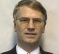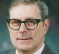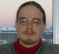## How to test my FFT implementation?Started by 3 years ago9 replieslatest reply 3 years ago1712 views

Currently what I do is generate random floating point values, feed it to my implementation and fftw simultaneously and check if any bin has deviation beyond a threshold (threshold = 0.005). The tolerance is there to compensate for floating point math inaccuracies. With this setup, my tests seem to pass. Is this enough though? I am new to dsp and I don't know how dsp code is tested. Any help would be highly appreciated.

Here's the repository if someone wants to see some actual code: https://github.com/devsh0/fft-playground/blob/mast...

I apologize for not making everything clear in the question. My implementation is a radix-2 real only dit-fft. I expect N, the input
size, to be a power of 2. Furthermore, I am taking FFTs of length
ranging between 2^2 to 2^16,  each sample array containing randomly
generated double precision floating point values. I have observed that
input sizes up to 2^17 don't cause errors greater in magnitude than .005 when compared to fftw's output for the same input sequence. I will run more tests suggested here on my implementation of the FFT. Thank you all for your submissions.

[ - ]Instead of random, use sine wave data.  Combine 2 different frequencies 90 degrees offset from each other and centered on your bins - you should get one real and one imaginary full max result with all other bins zero.  Then shift the frequencies to be at the edge of a bin.  You should get 2 bins next to each other with little bleed on other neighbors.

Comparing to fftw is valid - but comparing to theory is good too.  If your results don't give what you expect you can learn something.

[ - ]That's not a very thorough test, as many processes will produce constrained random output for random input.  So you could have something other than an FFT and get the same result.

If it produces Fourier Transform Pairs across input/output, then it is likely to be an FFT.

[ - ]Random noise can produce wildly random results (well, because..).

It's a reasonable approximation but the statistics aren't always as good is hoped.

You didn't say if this was complex I/Q input data or purely real values. For purely real values you can check the aliased complex conjugates match.

You could time reverse the sequence (phase reversal), or could use a linear time shift and check that the phases shift, but not the amplitudes.

[ - ]This is a fairly complicated answer. Let me apologize that I don't know the programming language for your testing code.

My first concern was that I don't know what FFT size N you are testing. Some FFT programs are limited to data sizes which are powers of two. Some are limited to composite N. Some (which I invented) can work for prime N and still do better than time O(N^2). Obviously, you have to test your program for all possible intended values of N.

We don't know why you developed your own FFT implementation. Was it just as a learning experience? Did you want more speed, or a program requiring less memory, or a program which could use some special novel hardware feature, etc.?

You have chosen FFTW as your comparison standard. It has been around long enough that it is probably bug-free. But is it the best standard for accuracy? The people who developed that program had the goal of writing the algorithm that would run fastest. We have to assume that they gave up some other optimizations for that. Certainly FFTW is not the shortest program. Has it compromised accuracy for speed? [I don't know why this paragraph isset in a different font?]

Some FFT algorithms I worked on were intended to access data arrays too large to fit into random access memory. At least in the distant past, large external memories were sequential storage, like tapes or rotating drums or disks. I don't know if FFTW would be able to do that and still be fast. I even designed one FFT algorithm to accept analog samples, using a charged coupled device processor, essentially an analog shift register. Certainly that CCD processor was less accurate than one using floating point arithmetic - just getting the original data accurate enough would have failed a test like yours.

Looking at some of the other replies, there's a common theme of trying to compare the result by using a different standard. For example, you could try a variety of inputs which have known DFTs, or DFTs which you could compute by a different algorithm. Thus, for example, you could take input x(n) and compute output X(k) and then see if input X*(n) gives you output (1/N) x(k).

Also, consider using a double precision version of FFTW and comparing its output with the output of your own single precision program. Then, instead of using a fixed tolerance of .005, you could compute a histogram of actual errors. That might point out some peculiarities.

Also, you would usually expect any reasonable FFT algorithm to produce negligible comparision error for bin 0, bin N/2, bin N/4 and bin 3N/4, especially for fixed point programs, because these bin numbers use no multiplications, only additions and subtractions.

Sorry for a long and complex answer. In fact, though, unless you have lots of time, you can be pretty confident that you've done enough already.

[ - ]Bittersweet,

You have to perform Three tests. Each is very telling.

test 1. Impulse response

in an array 0 to N-1,

place the largest amplitude impulse in address +1 This this the largest negative number.

Its FFT is a single cycle of a cosine in the real array and a sing cycle of a sine in the imaginary array.

test 2. Single complex sinusoid;

place one cycle of maximum amplitude cosine in real array and maximum amplitude sine in imaginary array.

Its FFT is a single maximum amplitude impulse in address 1. All other addresses are supposed to be be zero, but will not be due to finite arithmetic. You will see your arithmetic noise floor.

test 3. maximum amplitude single even symmetric rectangle sign(cos(2*pi*n/N)) in real array and single odd symmetric rectangle sign(sin(2*pi*n/N)) in imaginary array.

This is the most stressful test of your scaling algorithm in the FFT code. Fundamental term is (4/pi)*N_amp*N_xfm ... A butterfly can have a larger gain than 2 per pass. This will detect an underestimate of growth (by 4/pi).

Advice,  don't do initial tests with random data.. need test that has known answer so you can verify its correctness or lack of correctness by inspection.

The scaling in the FFT is the worst source of computer noise, insufficient number of bits in trig table is the second bad guy! need more trig table bits than data bits!

fred h

[ - ]You can give real only cosine waves from f0=Fs/N to Fs/N*N/2 with imaginary zeros as input.You will see +Ve going impulse pairs at Fs/N Fs/N*(N-1) ,Fs/N*2&Fs/N(N-2) etc in real part with imaginary part=0.Next you may give sine waves similar frequencies.You will see real part =0.Imaginary part Fs/N will be +Ve going impulse and Fs/N*(N-1) negative going impulse.All other bins=0 except 2 bins.You may do for all up to Nyquist.

Next apply frequencies between Fs/N and Fs/N*2 exactly in the centre for both cosine and sine.Due to FFT leakage you will see for example peaks at Fs/N *Fs/N*2,Fs/N(N-1) &Fs/N(N-2) .Small valuse will be for other bins.This in between frequencies verify for cosine and sine.

[ - ]1. You can perform FFT twice after that you will get the original inverted data (with indeces 0,N-1,...,2,1)
2. You can perform FFT from the identity matrix (N signal like x_j(i-j)=1 if i=j, otherwise 0) after that you will get the matrix of Fourier (with exp(2*\pi*i/N)^{k*j}, k,j=0:N-1)
[ - ]hi,

test with rand is required. additionally include different tones to cover Nyquist range. test using a chirp is also useful.

the easiest way to test is to take fft() and then take ifft() and compare the SNR. repeat for different tones. you can also include impulse or step in the test cases.

most often, you can covert your fft to ifft easily so that you can use for testing. best approach would be to save your fft output and use a reference ifft, such as from matlab of some open source.

if your fft is in fixed point include input cases with differnt ranges - both high and low.

hope it helps.

chalil

[ - ]Can use sine data (as drmike suggested).  Here's an implementation for filling input data to an FFT, to place a single peak in the bin and amplitude of your choosing:

uint32_t idx;

float64_t tmp_f0;

for ( idx = 0; idx < FFT_TEST_FFT_SIZE; idx++ )

{

tmp_f0 = FFT_TEST_FREQ;

tmp_f0 *= TWO_PI;

tmp_f0 *= ( (float64_t)idx / (float64_t)( FFT_TEST_SAMPLE_RATE ) );

tmp_f0 = FFT_TEST_FFT_AMP * (float64_t)cos( tmp_f0 );

fft_in_buf[ idx ] = tmp_f0;

}

With these example defines:

#define FFT_TEST_FFT_SIZE           128U

#define FFT_TEST_BIN_SIZE           2.0

#define FFT_TEST_FREQ               20.0

#define FFT_TEST_FFT_AMP            8.0

#define FFT_TEST_FFT_SCALE          ( 2.0 / FFT_TEST_FFT_SIZE )

#define FFT_TEST_SAMPLE_RATE     ( FFT_TEST_FFT_SIZE *FFT_TEST_BIN_SIZE )

after taking the power spectrum of the FFT output, and scaling by FFT_TEST_FFT_SCALE, we will see a peak of amplitude 8 at the 11th bin of a 128 point FFT, where bin 0 is DC (and nothing in any of the other bins).